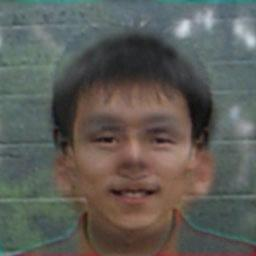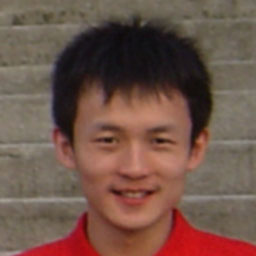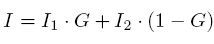## Hybrid Images

[Home]

### The Idea

This interesting hybrid-image idea was published in Siggraph'06 by people from MIT and U Glasgow,
Hybrid Images - A. Oliva, A. Torralba, P.G. Schyns, ACM Transactions on Graphics, vol. 25-3, pages 527-530, 2006.

The key is to combine the high frequency components of one image and the low frequency components of another image into a single image.

### My Implementation

This synthesyzed hybrid image looks like one person from close up and looks like another person from five meters away.### The Procedure

• Prepare two images that are roughly aligned.It's me Photo Curtersy by Changyin Zhou
• Generate the output hybrid-image using the following equation in Fourier domain,where G is a Gaussian Filter.

### The Code

• To have a try, here is a simple Matlab implementation, hybridimg.m.
 function hybridimg(ImgFile1, ImgFile2, ImgFileOut) % Hybrid Image Generator % Usage: hybridimg(, , );    radius = 13; % Param of the Gaussian radius    I1 = imread(ImgFile1);    I2 = imread(ImgFile2);    I1_ = fftshift(fft2(double(I1)));    I2_ = fftshift(fft2(double(I2)));    [m n z] = size(I1);    h = fspecial('gaussian', [m n], radius);    h = h./max(max(h));    for colorI = 1:3       J_(:,:,colorI) = I1_(:,:,colorI).*(1-h) + I2_(:,:,colorI).*h;    end    J = uint8(real(ifft2(ifftshift(J_))));    imwrite(J, ImgFileOut); end

[Go to my Homepage]
Page last updated: December 18, 2007 10:05 AM